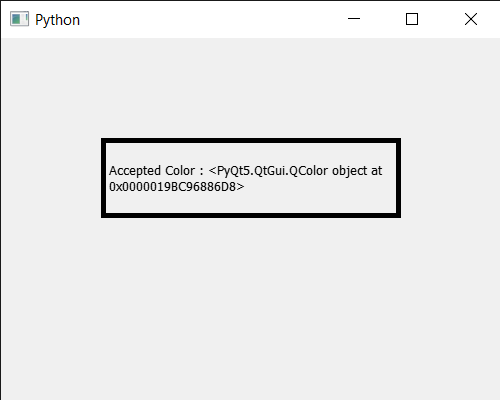# PyQt5 QColorDialog – Rejecting the color

• Last Updated : 24 Jun, 2020

In this article we will see how we can reject the color in the QColorDialog widget. Hides the modal dialog and sets the result code to Rejected., it is same when user press ‘Cancel’ button.

This method call the finished signal

In order to do this we use `reject` method with the QColorDialog object

Syntax : dialog.reject()

Argument : It takes no argument

Return : It returns None

Below is the implementation

 `# importing libraries``from` `PyQt5.QtWidgets ``import` `*` `from` `PyQt5 ``import` `QtCore, QtGui``from` `PyQt5.QtGui ``import` `*` `from` `PyQt5.QtCore ``import` `*` `import` `sys`` ` ` ` `class` `Window(QMainWindow):`` ` `    ``def` `__init__(``self``):``        ``super``().__init__()`` ` `        ``# setting title``        ``self``.setWindowTitle(``"Python "``)`` ` `        ``# setting geometry``        ``self``.setGeometry(``100``, ``100``, ``500``, ``400``)`` ` `        ``# calling method``        ``self``.UiComponents()`` ` `        ``# showing all the widgets``        ``self``.show()`` ` ` ` `    ``# method for components``    ``def` `UiComponents(``self``):`` ` `        ``# creating a QColorDialog object``        ``dialog ``=` `QColorDialog(``self``)`` ` `        ``# setting current color to the dialog``        ``dialog.setCurrentColor(Qt.red)`` ` `        ``# accepting the color``        ``# now color will become selected one``        ``dialog.accept()`` ` `        ``# rejecting the color``        ``dialog.reject()`` ` `        ``# executing the dialog``        ``# dialog.exec_()`` ` ` ` `        ``# creating label``        ``label ``=` `QLabel(``"GeeksforGeeks"``, ``self``)`` ` `        ``# setting geometry to the label``        ``label.setGeometry(``100``, ``100``, ``300``, ``80``)`` ` `        ``# making label multi line``        ``label.setWordWrap(``True``)`` ` `        ``# setting stylesheet of the label``        ``label.setStyleSheet(``"QLabel"``                            ``"{"``                            ``"border : 5px solid black;"``                            ``"}"``)`` ` `        ``# getting the selected color``        ``color ``=` `dialog.selectedColor()`` ` `        ``# setting graphic effect to the label``        ``graphic ``=` `QGraphicsColorizeEffect(``self``)`` ` `        ``# setting color to the graphic``        ``graphic.setColor(color)`` ` `        ``# setting graphic to the label``        ``label.setGraphicsEffect(graphic)`` ` `        ``# setting text to the label``        ``label.setText(``"Accepted Color : "` `+` `str``(color))`` ` ` ` ` ` `# create pyqt5 app``App ``=` `QApplication(sys.argv)`` ` `# create the instance of our Window``window ``=` `Window()`` ` `# start the app``sys.exit(App.``exec``())`

Output :Note : In this implementation we didn’t execute the color dialog because we set the current color and accept the color programmatically will make it selected color instead of white which is default but then the reject method get called which will remove the color so the color becomes black

My Personal Notes arrow_drop_up